The current in the adjoining circuit will be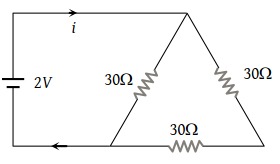(1) $\frac{1}{45}ampere$

(2) $\frac{1}{15}ampere$

(3) $\frac{1}{10}ampere$

(4) $\frac{1}{5}ampere$

Concept Questions :-

Combination of resistors
High Yielding Test Series + Question Bank - NEET 2020

Difficulty Level:

The reading of the ammeter as per figure shown is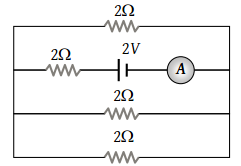(1) $\frac{1}{8}A$

(2) $\frac{3}{4}A$

(3) $\frac{1}{2}A$

(4) 2 A

Concept Questions :-

Combination of resistors
High Yielding Test Series + Question Bank - NEET 2020

Difficulty Level:

Three resistors each of 2 ohm are connected together in a triangular shape. The resistance between any two vertices will be

(1) 4/3 ohm

(2) 3/4 ohm

(3) 3 ohm

(4) 6 ohm

Concept Questions :-

Combination of resistors
High Yielding Test Series + Question Bank - NEET 2020

Difficulty Level:

There are n similar conductors each of resistance R. The resultant resistance comes out to be x when connected in parallel. If they are connected in series, the resistance comes out to be :

(1) x/n2

(2) n2x

(3) x/n

(4) nx

Concept Questions :-

Combination of resistors
High Yielding Test Series + Question Bank - NEET 2020

Difficulty Level:

Equivalent resistance between A and B will be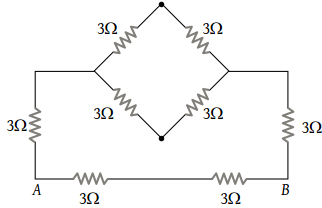(1) 2 ohm

(2) 18 ohm

(3) 6 ohm

(4) 3.6 ohm

Concept Questions :-

Combination of resistors
High Yielding Test Series + Question Bank - NEET 2020

Difficulty Level:

A current of 2 A flows in a system of conductors as shown. The potential difference $\left({V}_{A}-{V}_{B}\right)$ will be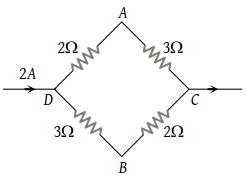(1) +2 V

(2) +1 V

(3) –1 V

(4) –2 V

Concept Questions :-

Kirchoff's voltage law
High Yielding Test Series + Question Bank - NEET 2020

Difficulty Level:

Referring to the figure below, the effective resistance of the network is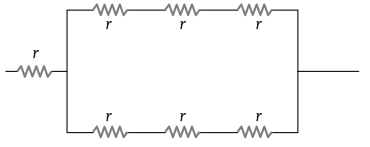(1) 2r

(2) 4r

(3) 10r

(4) 5r/2

Concept Questions :-

Combination of resistors
High Yielding Test Series + Question Bank - NEET 2020

Difficulty Level:

Two resistances are joined in parallel whose resultant is $\frac{6}{8}$ ohm. One of the resistance wire is broken and the effective resistance becomes 2Ω. Then the resistance in ohm of the wire that got broken was

(1) 3/5

(2) 2

(3) 6/5

(4) 3

Concept Questions :-

Combination of resistors
High Yielding Test Series + Question Bank - NEET 2020

Difficulty Level:

The equivalent resistance of resistors connected in series is always :

(1) Equal to the mean of component resistors

(2) Less than the lowest of component resistors

(3) In between the lowest and the highest of component resistors

(4) Equal to the sum of component resistors

Concept Questions :-

Combination of resistors
High Yielding Test Series + Question Bank - NEET 2020

Difficulty Level:

A cell of negligible resistance and e.m.f. 2 volts is connected to a series combination of 2, 3, and 5 $\Omega$. The potential difference in volts between the terminals of 3 $\Omega$ resistance will be :

(1) 0.6 V

(2) 2/3 V

(3) 3 V

(4) 6 V

Concept Questions :-

Grouping of cells